04 Mar
04Mar

As a professional supplier of insulating glass production equipment in the glass deep processing industry, Jinan Lijiang Automation Equipment Co., Ltd. will often be asked by customers about investment costs. Through the accumulation of years of business experience of our overseas business personnel, we can provide relevant answers to such questions A detailed summary was made. The following will give you a detailed introduction on how to calculate the production cost of one square meter of insulating glass, which is convenient for purchasers and friends who plan to enter the industry as an investment reference.

Simply put, the main raw materials for the production and processing of insulating glass: glass, aluminum spacers, molecular sieves, two-component AB glue, butyl glue, pins, etc. The main production costs of insulating glass include rent, labor, electricity, material loss, and so on.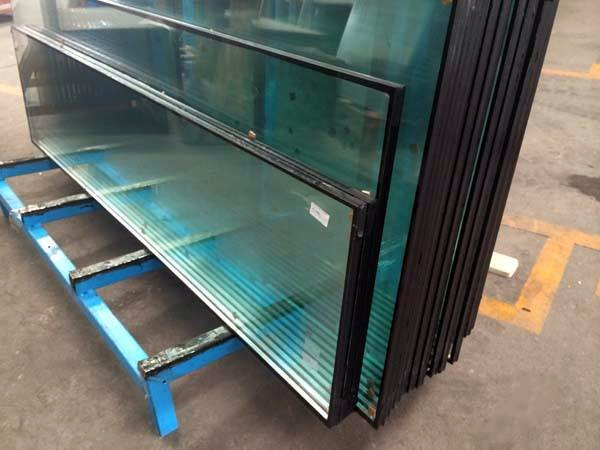Glass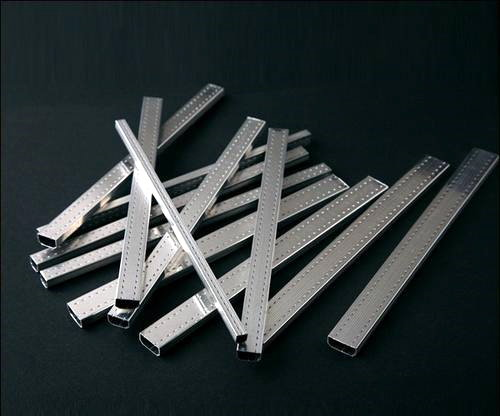Aluminum Spacers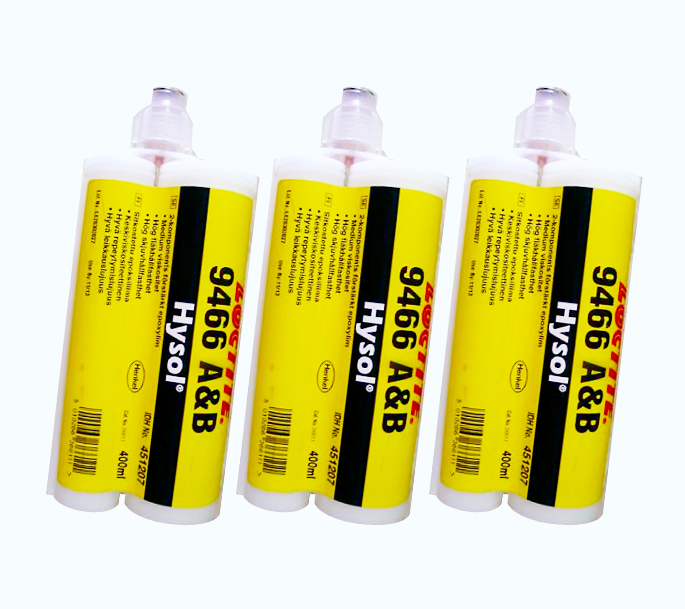Two-component AB Glue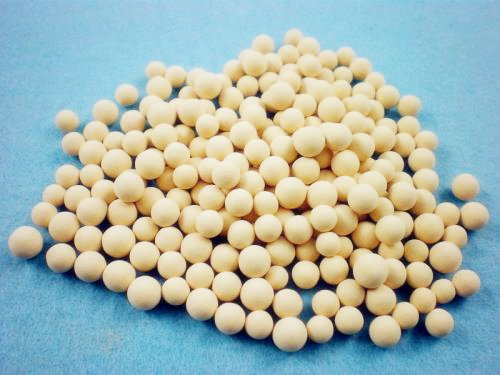Molecular Sieves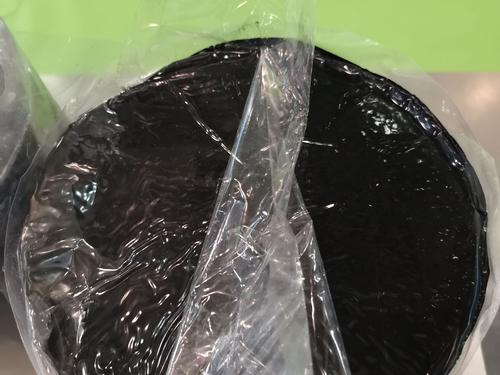Butyl Glue

In order to ensure the accuracy of the calculation results, the following three conditions are necessary for calculation:

①In order to ensure that the calculation results are not affected by other special factors, especially raw material price fluctuations, all raw materials are generally composed of three grades: high-grade, intermediate-grade, and low-grade. The prices of raw materials are generally distributed according to the grade, from high to low. When calculating the cost of raw materials, the raw materials of the middle grade and the middle price are used as the standard.

②For the convenience of the price calculation, all calculated prices are calculated at an exchange rate of 6.5 USD from RMB to USD.In the actual calculation, the unit of RMB "yuan" is used as the unit and finally converted into US dollar units.

So now start to calculate the production price of a piece of one square meter of insulating glass:

1. The glass is calculated based on the middle-grade glass price, 12.5 yuan per square meter. Taking hollow two-glass glass as an example, the price of two pieces of glass is 12.5*2=25 yuan.

2. The production cost of aluminum spacers is 160 yuan per pack. There are 90 aluminum spacers in a pack. An aluminum spacer is about 6 meters long. Then the price of one square meter of an aluminum spacer is 0.3 yuan. 0.3*4=1.2 yuan per square meter.

3. Molecular sieves are generally 4-8 yuan per kilogram, so take the mid-range molecular sieve 6 yuan per kilogram, and one square meter of insulating glass can be filled with molecular sieves during production, and molecular sieves cost about 0.5 yuan.

4. The market cost price of two-component AB glue is 10-14 yuan per kilogram, so one square meter needs 6 yuan AB glue.

5. The market cost price of butyl rubber is 14-16 yuan per kilogram, so one square meter of insulating glass can consume about 0.3 yuan of butyl rubber.

6. For the most basic consumable accessories such as pins, the actual processing cost can be ignored.

7. The cost of production such as rent, labor, material loss, etc., because labor costs and rent costs in each country and region are different, so this factor is temporarily not included.

Therefore, the production cost of one square meter of insulating glass is 25+1.2+0.5+6+0.3=33 yuan and then calculated according to the current spot exchange rate of RMB to USD 6.5 USD,

33 ÷ 6.5=5.077 USD / Piece

Due to the different thickness of the glass, the width of the aluminum spacer, the filling volume of the molecular sieve, and the price of the sealant, the more accurate cost and profit of insulating glass need to be calculated according to the actual situation.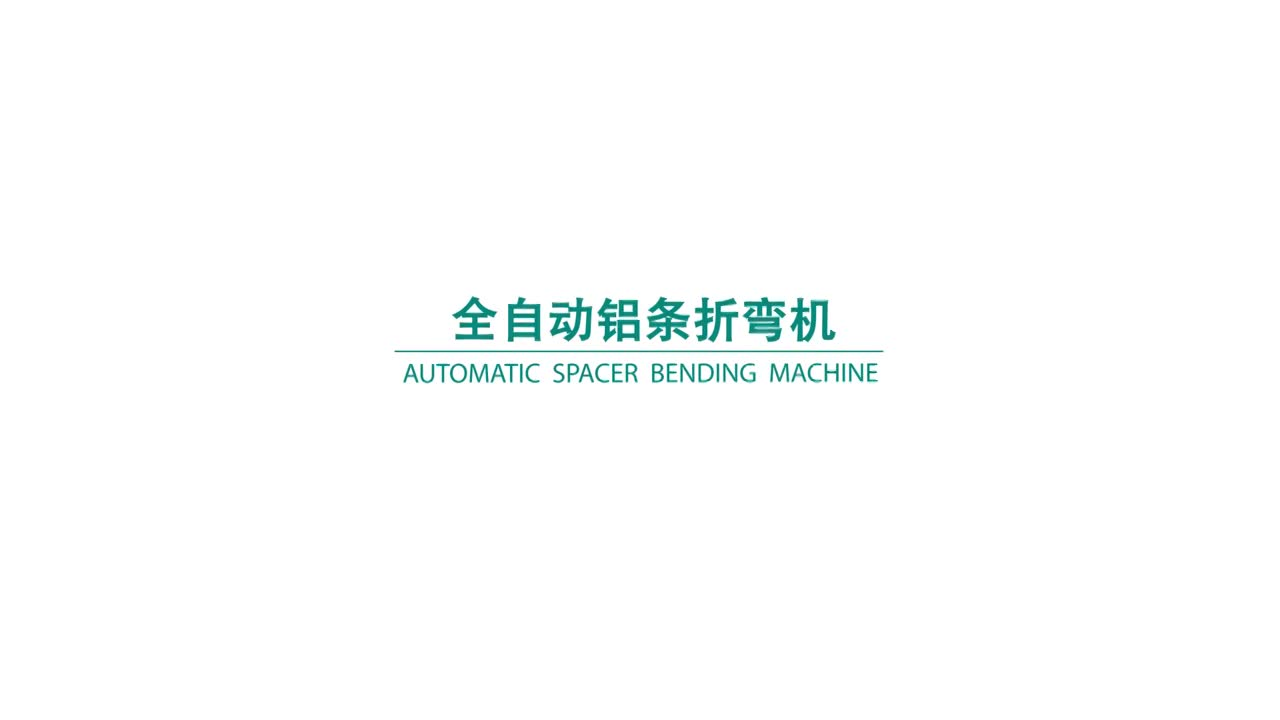The above is the cost of producing one square meter of insulating glass based on years of experience in the glass deep processing industry by Jinan Lijiang Automation Equipment Co., Ltd., please correct me if there is any error. If readers are interested in how to improve the production efficiency of glass deep processing, they can learn about our company's related automation equipment for glass deep processing.